# The interval versions of the Kalman filter and the EM algorithm

## Abstract

In this paper, we study state space models represented by interval parameters and noise. We introduce an interval version of the Expectation Maximization (EM) algorithm for the identification of the interval parameters of the system. We also introduce a suboptimal interval Kalman filter for the identification and estimation of the state vectors. The work requires the introduction of the concept of interval random variables which we also include in this work together with a study of their interval statistical properties such as expectation, conditional expectation and variance. Although the interval Kalman filter introduced here is suboptimal, it successfully recovers the state vectors to a high precision in the simulation examples we have run.

## 1 Introduction

In a state space model, some parameters of the system such as the coefficient matrices may not be precisely known or they gradually change with time. One way to account for these uncertainties is to allow such parameters to be represented by interval entities. The question then arises as to how to extend identification and estimation techniques to interval settings.

To our knowledge, no attempt has been made so far to extend identification techniques such as the EM algorithm to interval state space models. In this work, we give one such an extension.

In the existing literature, an optimal interval Kalman filter was attempted in . That attempt suffered from the lack of proper definitions and rigorous treatment. The idea in  was to replace the interval system setting with the ‘worst case inversion’ while keeping everything else unchanged. So, the ultimate treatment in  amounts to the application of the traditional Kalman filter to the system representing the worst case scenario. This way the authors were able to avoid the difficulties that arise when dealing with interval arithmetic and concepts. On the other hand, this algorithm cannot be called optimal and the concept of the optimal interval Kalman filter remains an open question.

In our work, we introduce a spacial interval arithmetic that always produces results that are smaller (in the sense that it is contained) than the traditional interval arithmetic [2, 3]. This arithmetic enables the extension of the Kalman filter as well as the EM algorithm to interval setting in a true sense. In our restricted interval arithmetic, the interval Kalman filter we introduce here is optimal. However, with respect to the more general interval arithmetic, our interval Kalman filter is suboptimal.

## 2 A special interval arithmetic

We introduce a special set of interval operations that will enable the extension of the usual linear system concepts to the interval setting in a seamless manner. The more general definitions of the interval operations can be found in . The arithmetic introduced here avoids such vague terms as ‘interval extension’, ‘inclusion function’, determinants etc. that have been used in the literature [1, 46].

All the interval operations adopted in this work stem from the view of an interval as a set of convex combinations of its endpoints:

$I=[a,b]= { x α = ( 1 − α ) a + α b : α ∈ [ 0 , 1 ] } .$

Definition 1 Suppose $I=[a,b]$, $J=[c,d]$ are intervals and $•∈{+,−,∗,÷}$. Define the following interval operations:

$I•J= { x α • y α : α ∈ [ 0 , 1 ] , x α ∈ I , y α ∈ J } ,$

with the usual restriction $0∉J$ if • = ÷.

Observe that all operations in Definition 1 result in intervals since they can be regarded as continuous functions defined on the unit interval $[0,1]$. For example, a typical element in $I∗J$ is $( 1 − α ) 2 ac+α(1−α)(ad+bc)+ α 2 bd$ which is a continuous function of α. The operations in Definition 1 give similar results to the usual interval operations as given in  when $•∈{+,−}$, but generally they give only subintervals if $•∈{∗,÷}$. For example, if $I=[−2,2]$, then $I∗I=[0,4]$ according to Definition 1, while the usual definition in  gives $I∗I=[−4,4]$.

The operations in Definition 1 are associative: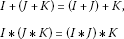and distributive:

$I∗(J+K)=I∗J+I∗K.$

For example, distributivity is shown as follows:

$I ∗ ( J + K ) = { I α ( J + K ) α : α ∈ [ 0 , 1 ] } = { I α ( J α + K α ) : α ∈ [ 0 , 1 ] } = { I α J α + I α K α : α ∈ [ 0 , 1 ] } = { ( I J ) α + ( I K ) α : α ∈ [ 0 , 1 ] } = { ( I J + I K ) α : α ∈ [ 0 , 1 ] } = I J + I K .$

These two properties, which are missing in the usual interval operations, will enable the extension of many results from usual state space models to interval state space models. On the other hand, these definitions were motivated by our attempt to arrive at a definition of interval random variables and investigate the corresponding statistical properties. We feel that they are the natural ones to handle interval systems. This feeling is reassured by the numerical results we obtained in the simulation examples (see Section 5). While we expected to obtain a construction of a suboptimal interval Kalman filter, the constructed filter was actually able to recover the exact simulated intervals rather than subintervals.

Interval vectors and matrices are defined similarly:

• A vector $v∈ IR n$ is defined as

$v=[a,b]= { x α = ( 1 − α ) a + α b : α ∈ [ 0 , 1 ] } ,$

where

$a,b∈ R n ,a≤b,$

and the inequality holds componentwise.

• A matrix $A∈ IR n × n$ is defined as

$A=[A,B]= { X α = ( 1 − α ) A + α B : α ∈ [ 0 , 1 ] } ,$

where

$A,B∈ R n × n ,A≤B,$

and the inequality holds componentwise.

Definition 2 Given a function $f: R k → R n$ and an interval vector $v∈ IR k$, we define

$f(v)= { f ( x α ) : α ∈ [ 0 , 1 ] } .$

If f is continuous, then $f(v)$ is an interval vector. All operations on functions are extended to interval settings in the same way. For example,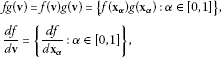provided that the involved operations make sense. In the same spirit, interval matrix operations are defined as follows:

• The interval determinant is defined by

$det(A)= { det ( X α ) : α ∈ [ 0 , 1 ] } .$
• The interval adjoint is defined by

$adj(A)= { adj ( X α ) : α ∈ [ 0 , 1 ] } .$
• The interval inverse is defined by

$A − 1 = { X α − 1 : α ∈ [ 0 , 1 ] } = { adj ( X α ) det ( X α ) : α ∈ [ 0 , 1 ] } = adj ( A ) det ( A ) .$

The continuous dependence of the determinant of a matrix on the elements of the matrix implies that all the above operations produce interval entities. Naturally, these special definitions produce results that are contained in the corresponding usual definitions. To give an example, we use the definition of the inverse interval matrix $A − 1$ according to :

$A − 1 = [ { X − 1 : X ∈ A } ] ,$

where $[S]$ is the smallest interval vector (matrix) containing $S$. If

$A=[ [ 2 ] [ − 1 , 1 ] [ − 1 , 1 ] [ 2 ] ],$

then

$S= { X − 1 : X ∈ A } = { [ 2 4 − r s − r 4 − r s − r 4 − r s 2 4 − r s ] : r , s ∈ [ − 1 , 1 ] } .$

One can show that the set of points in $R 2$ with coordinates equal to the first row of the elements of $S$ forms a polygonal (non-rectangular) region with vertices at $( 2 5 , 1 5 )$, $( 1 2 ,0)$, $( 2 3 , 1 3 )$, $( 2 5 ,− 1 5 )$, $( 2 3 ,− 1 3 )$. Thus,

$A − 1 =[S]=[ [ 2 5 , 2 3 ] [ − 1 3 , 1 3 ] [ − 1 3 , 1 3 ] [ 2 5 , 2 3 ] ].$

The inverse in our sense is

$A − 1 =[ [ 1 2 , 2 3 ] [ 0 , 1 3 ] [ 0 , 1 3 ] [ 1 2 , 2 3 ] ].$
• Suppose that $A − 1$ exists. We define the solution of the interval linear system $AX=b$ to be

$X= { X ∈ R n : A α X = b α , α ∈ [ 0 , 1 ] } .$

Clearly, X is an interval vector. The usual definition is

$S= { X ∈ R n : ∃ A ∈ A , b ∈ b , A X = b } .$

Obviously, our definition produces a smaller interval vector. In fact, if $A − 1$ exists in the sense of , then

$X⊂S⊂[S]⊂ A − 1 b.$

The last inclusion holds because if $X∈S$, then there is an $A∈A$ and a $b∈b$ with $AX=b$. Then $X= A − 1 b∈ A − 1 b⊂ A − 1 b$. Thus, $S⊂ A − 1 b$. Noting that $A − 1 b$ is an interval vector and $[S]$ is minimal, we get that $[S]⊂ A − 1 b$.

For the rest of this paper, we will use the special interval operations defined above.

Finally, for error estimates, we need to introduce the distance between two intervals $I=[a,b]$, $J=[c,d]$. This is defined by

$q(I,J):=max { | a − c | , | b − d | } .$

The map q defines a metric in IR.

## 3 Interval random variables

We begin by discussing the measurability of set-valued maps and then introduce the definition of an interval random variable. The basic definitions and more details can be found in . A measurable space $(Ω,A)$ consists of a basic set Ω together with a σ-algebra $A$ of subsets of Ω called measurable sets. Here, we consider closed convex value set-valued maps $F:Ω⇉ R k$, i.e., $F(ω)$ is a closed convex subset of $R k$ for each $ω∈Ω$. This is the case when F is interval valued. The latter notion means that for each $ω∈Ω$, the components of $F(ω)$ are closed intervals in .

We first define what it means for a set-valued map to be measurable. Recall that the inverse image of a set $S⊂ R k$ under the set-valued map F is defined by

$F − 1 (S)= { ω ∈ Ω : F ( ω ) ∩ S ≠ ∅ } ,$

and that the graph of F (denoted by $G F$) is defined by

$G F = { ( ω , y ) : ω ∈ Ω , y ∈ F ( ω ) } .$

Definition 3 Let $(Ω,A)$ be a measurable space and $F:Ω⇉ R k$ be a set-valued map. F is called measurable if the inverse image of each open set is a measurable set: if $O⊂ R k$ is open, then $F − 1 (O)∈A$.

We are now in a position to introduce the definition of interval random variables and interval stochastic processes.

Definition 4 Let $(Ω,S,P)$ be a probability space. An interval-valued map $X:Ω⇉ R k$ is called an interval random variable if

1. 1.

X is measurable, and

2. 2.

the function $x↦ p x$ is continuous on X, where $p x$ is the probability density function for the random variable x.

An interval stochastic process is an indexed set of interval random variables.

The probability density function $p X$ is then the interval-valued function

$p X ={ p x :x∈X}.$

In order to study the expectations and variances of interval random variables, we need to discuss first the integral of set-valued maps and, in particular, interval-valued maps. The discussion begins with the notion of measurable selections.

Definition 5 Let $(Ω,A)$ be a measurable space and $F:Ω⇉ R k$ be a measurable set-valued map. A measurable selection of F is a measurable map $f:Ω→ R k$ satisfying $f(ω)∈F(ω)$ for each $ω∈Ω$.

It is well known that every measurable set-valued map has at least one measurable selection . Furthermore, we have the following equivalences .

Theorem 6 Let $(Ω,A)$ be a measurable space and denote by the σ-algebra of Borel sets in $R k$. Let $F:Ω⇉ R k$ be a set-valued map. The following are equivalent.

1. 1.

F is measurable.

2. 2.

$G F ∈A⊗B$.

3. 3.

$F − 1 (B)∈A$ for every $B∈B$.

4. 4.

There exists a sequence of measurable selections ${ f n } n = 1 ∞$ of F such that

$F(ω)= ⋃ n ≥ 1 f n ( ω ) ¯$

for each $ω∈Ω$.

A countable family of measurable selections satisfying the last property is called dense.

Let $F:Ω⇉ R k$ be an interval-valued map. We define the two special functions $l F$ and $r F$ such that $l F (ω)=a(ω)$ and $r F (ω)=b(ω)$, where $F(ω)=[a(ω),b(ω)]$ for each $ω∈Ω$. The next lemma shows that $l F$ and $r F$ are measurable selections of F when the latter is measurable.

Lemma 7 Let $F:Ω⇉ R k$ be a measurable interval-valued map. Then the point functions $l F$ and $r F$ are measurable selections of F.

Proof Choose a sequence of measurable selections ${ f n } n = 1 ∞$ of F such that

$F(ω)= ⋃ n ≥ 1 f n ( ω ) ¯ .$

Then $l F (ω)= inf n ≥ 1 f n (ω)$ and $r F (ω)= sup n ≥ 1 f n (ω)$ (here the inf and sup operations are taken componentwise). Since the inf and the sup operators preserve measurability, we see that the functions $l F$ and $r F$ are measurable selections of F. □

Example Let $Ω=[1,∞)$ and define $F:Ω⇉R$ by

$F(t)= [ t , t + 1 t ] .$

Let ${ r n } n = 1 ∞$ be an enumeration of the rational numbers in the interval $[0,1]$, and let us assume that $r 1 =1$, $r 2 =0$. Define $f n :[1,∞)→R$ by

$f n (t)= r n t+(1− r n ) ( t + 1 t ) .$

Thus, $l F (t)=t= f 1 (t)$ and $r F (t)=(t+ 1 t )= f 2 (t)$. For every $t∈[1,∞)$, the set ${ r n t + ( 1 − r n ) ( t + 1 t ) } n = 1 ∞$ is dense in the interval $[t,t+ 1 t ]$. Thus, $F(t)= ⋃ n ≥ 1 f n ( t ) ¯$.

Now suppose that $(Ω,A,μ)$ is a measure space and $F:Ω⇉ R k$ is a set-valued map. A measurable selection f of F is an integrable selection if f is integrable with respect to the measure μ. The set of all integrable selections of F will be denoted by . The map F is called integrably bounded if there exists a μ-integrable function $g∈ L 1 (Ω;R,μ)$ such that $F(ω)⊂g(ω)B$ for μ-almost every $ω∈Ω$. Here, B denotes the unit ball in $R k$. In this case, every measurable selection f of F is also an integrable selection since $f(ω)∈F(ω)⊂g(ω)B$ implies that $∥f(ω)∥≤|g(ω)|$, where $∥⋅∥$denotes the Euclidean norm on $R k$.

Definition 8 The integral of a set-valued map F is defined to be the set of integrals of integrable selections of F. That is,

$∫ Ω Fdμ= { ∫ Ω f d μ : f ∈ F } .$
(1)

We shall say that F is integrable if every measurable selection is integrable.

We have the following immediate properties: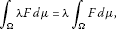(2)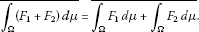(3)

Lemma 9 Let $F:Ω⇉ R k$ be an interval-valued map. If $l F$ and $r F$ are integrable, then F is integrable and

$∫ Ω F d μ = [ ∫ Ω l F d μ , ∫ Ω r F d μ ] = { ∫ Ω f α d μ : f α = α l F + ( 1 − α ) r F , α ∈ [ 0 , 1 ] } .$

Proof The first equality is shown as follows. Since for every $ω∈Ω$ and every integrable selection f of F we have $l F (ω)≤f(ω)≤ r F (ω)$,

$∫ Ω l F (ω)dμ≤ ∫ Ω f(ω)dμ≤ ∫ Ω r F (ω)dμ.$

Therefore,

$∫ Ω Fdμ⊆ [ ∫ Ω l F d μ , ∫ Ω r F d μ ] .$

On the other hand, let $θ∈[ ∫ Ω l F dμ, ∫ Ω r F dμ]$. We may write $θ=(1−α) ∫ Ω l F dμ+α ∫ Ω r F dμ$ for some $α∈[0,1]$. Then

$θ = ∫ Ω ( ( 1 − α ) l F + α r F ) d μ = ∫ Ω f α d μ ,$

where $f α =(1−α) l F +α r F$. Hence, $θ∈ ∫ Ω Fdμ$.

The second equality is an immediate consequence of this. □

It will always be assumed that both $l F$ and $r F$ are integrable.

Example Let Ω and F be defined as in the previous example. Let μ be the measure defined by

$dμ= 1 t 3 dt.$

Then

$∫ Ω Fdμ= [ ∫ 1 ∞ l F ( t ) d μ , ∫ 1 ∞ r F ( t ) d μ ] = [ 1 , 4 3 ] .$

In view of (3), we have the following corollary.

Corollary 10 Let $F 1 , F 2 :Ω⇉ R k$ be integrable interval-valued maps. Then

$∫ Ω ( F 1 + F 2 ) d μ = ∫ Ω F 1 d μ + ∫ Ω F 2 d μ = [ ∫ Ω l F 1 d μ , ∫ Ω r F 1 d μ ] + [ ∫ Ω l F 2 d μ , ∫ Ω r F 2 d μ ] = [ ∫ Ω ( l F 1 + l F 2 ) d μ , ∫ Ω ( r F 1 + r F 2 ) d μ ] .$

Let $(Ω,S,P)$ be a probability space, and let $Z:Ω⇉ R k$ be an interval random variable. We have

$Z(ω)= [ l Z ( ω ) , r Z ( ω ) ] = { z α : = ( 1 − α ) l Z ( ω ) + α r Z ( ω ) : α ∈ [ 0 , 1 ] } .$

We shall say that Z is normally distributed if each $z∈Z$ is normally distributed. An interval stochastic process ${ Z t } t ∈ T$ will be called normally distributed if for each $t∈T$, $Z t$ is normally distributed.

Let Z be an interval random variable. Then for each $z∈Z$,

$p l z ≤ p z ≤ p r z .$

By the continuity of $z↦ p z$,

$p Z =[ p l z , p r z ].$

This means that

$l p Z = p l z , r p Z = p r z .$

Guided by this and Lemma 9, we can define the interval expectation of the interval random variable Z as follows.

Definition 11 The interval expectation of an interval random variable Z is defined as

$E(Z)= [ E ( l Z ) , E ( r Z ) ] .$

This definition coincides with Definition 2 since

$[ E ( l Z ) , E ( r Z ) ] = { α E ( l Z ) + ( 1 − α ) E ( r Z ) : α ∈ [ 0 , 1 ] } = { E ( α l Z + ( 1 − α ) r Z ) : α ∈ [ 0 , 1 ] } = { E ( z α ) : α ∈ [ 0 , 1 ] } .$

It should also be noted that the expectation of a vector random variable is the vector of expectations of its components.

It follows from equations (2) and (3) that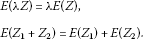Also, if $I=[a,b]$ and Z is an interval random variable, then

$E ( I Z ) = { E ( t α z α ) : α ∈ [ 0 , 1 ] } = { t α E ( z α ) : α ∈ [ 0 , 1 ] } = I ∗ { E ( z α ) : α ∈ [ 0 , 1 ] } = I E ( Z ) .$

The same is true if I is an interval vector and Z is an interval random variable.

More generally, if A is a $k×k$ interval matrix and if its columns are denoted by the interval vectors $A 1 , A 2 ,…, A k$, then

$E ( AZ ) = E ( ∑ j = 1 k A i Z j ) = ∑ j = 1 k E ( A i Z j ) = ∑ j = 1 k A i E ( Z j ) = A E ( Z ) .$

To introduce covariance of two interval random variables Y, Z, we need to assume that the function $(x,y)↦ p x , y$ is continuous on $Y×Z$. Here, $p x , y$ is the joint probability density function of the two random variables x, y.

Definition 12 The interval covariance of two interval random variables Y, Z is defined as

$Cov(Y,Z)= { Cov ( y α , z α ) : α ∈ [ 0 , 1 ] } .$

To see that $Cov(Y,Z)$ is an interval, note that

$Cov ( Y , Z ) = { Cov ( ( 1 − α ) l Y + α r Y , ( 1 − α ) l Z + α r Z ) : α ∈ [ 0 , 1 ] } = { ( 1 − α ) 2 Cov ( l Y , l Z ) + α ( 1 − α ) Cov ( l Y , r Z ) + α ( 1 − α ) Cov ( r Y , l Z ) + α 2 Cov ( r Y , r Z ) : α ∈ [ 0 , 1 ] } .$

If $Y=Z$, we get the definition of the variance of an interval random variable Z as

$Var ( Z ) = { Var ( z α ) : α ∈ [ 0 , 1 ] } = { ( 1 − α ) 2 Var ( l Z ) + 2 α ( 1 − α ) Cov ( l Z , r Z ) + α 2 Var ( r Z ) : α ∈ [ 0 , 1 ] }$

which is also an interval. Elementary calculus considerations reveal that

$Var(Z)= [ a b − c 2 a + b − 2 c , max { a , b } ] ,$

where $a=Var( l Z )$, $b=Var( r Z )$, $c=Cov( l Z , r Z )$. This last equation provides a formula for computing the interval $Var(Z)$.

For interval random vectors, the above definitions hold componentwise.

The two interval random variables Y, Z will be called uncorrelated if for each $y α ∈Y$, $z α ∈Z$, $y α$, $z α$ are uncorrelated. Therefore, Y, Z are uncorrelated if and only if $Cov(Y,Z)=$.

It is now straightforward to check the following theorem.

Theorem 13 Let $Y,Z∈ IR k$, $W∈ IR m$, $IR n$ be interval random vectors, and let $A∈ IR k ′ × k$, $B∈ IR m ′ × m$, $λ∈IR$, then

1. 1.

$Cov(λY,W)=λCov(Y,W)$,

2. 2.

$Cov(Y+Z,W)=Cov(Y,W)+Cov(Z,W)$,

3. 3.

$Cov(AY,BW)=ACov(Y,W) B T$.

The assumed continuous dependence of the probability density function (joint density function) on the random variable (variables) in an interval random variable (interval random variables) implies that the conditional probability density function is also continuous. This guarantees that the generalization of the conditional density function to the interval setting is always an interval.

Definition 14 The interval conditional expectation is defined as

$E ( Z | Y ) = { E ( z α | y α ) : α ∈ [ 0 , 1 ] } = { α E ( l Z | y α ) + ( 1 − α ) E ( r Z | y α ) : α ∈ [ 0 , 1 ] } .$

The following theorem is easily checked.

Theorem 15 For vector random variables X, Y, Z and interval matrix A of appropriate dimensions,

1. 1.

$E(X+Y|Z)=E(X|Y)+E(Y|Z)$,

2. 2.

$E(AY|Z)=AE(Y|Z)$.

## 4 The interval state space model

The interval state space model we will consider here is one of the form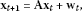(4)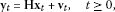(5)

where $A∈ IR k × k$, $H∈ IR p × k$ are interval matrices and $w t ∈ IR k$, $v t ∈ IR p$ are zero-mean Gaussian white-noise interval processes, with

$Cov ( [ w t v t ] , [ w s v s ] ) =[ Q 0 0 R ] δ t s ,$

while the initial state $x 0$ is assumed to be an interval random variable having zero-mean, interval variance matrix $Π 0$ and to be uncorrelated to ${ w t }$ and ${ v t }$ for all $t≥0$. The matrices $Q∈ IR k × k$, $R∈ IR p × p$ are also allowed to be interval matrices. For the time being, we assume that the matrices F, H, Q, R are known a priori. We thus have the properties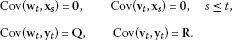For the state covariance matrix

$Π t =Cov( x t , x t ),$

we have the recursion

$Π t + 1 =A Π t A T +Q,t≥0$

with initial value $Π 0$.

### 4.1 The interval Kalman filter

In this section, we give a summary of the interval settings of the Kalman filter and the EM algorithm. The ground work that we did in the previous two section should reveal that it is possible to apply both methods to interval state space models. Let $Y s ={ y 1 , y 2 ,…, y s }$ be a sequence of interval measurements up to time s and let

$x t s =E( x t | Y s ).$

The expectation is a forecast for $s, a filtered value for $s=t$ and a smoothed value for $s>t$. The least square estimation error is defined by

$P t s =E ( ( x t − x t s ) ( x t − x t s ) T ) .$

The interval Kalman filter is defined in two main steps: estimation and forecast [9, 10]. The estimation step is given by

$x t t − 1 = Ax t − 1 t − 1 , P t t − 1 =A P t − 1 t − 1 A T +Q,$

and the forecast step is given by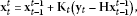(6)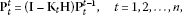(7)

where

$K t = P t t − 1 ( HP t t − 1 H T + R ) − 1$

is called the Kalman gain. The initial conditions are $x 0 0 = μ 0$ and $P 0 0 = Σ 0$.

The interval Kalman smoother, which is needed for the EM algorithm, is defined by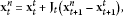(8)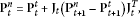(9)

where

$J t = P t t A T ( P t + 1 t ) − 1 .$

The initial conditions $x n n$ and $P n n$ in this case are found from (6) and (7). The EM algorithm also needs the so-called lag-one covariance smoother defined by

$P n , n − 1 n = ( I − K n H ) AP n − 1 n − 1 , P t , t − 1 n = P t t J t − 1 T + J t T ( P t + 1 , t n − AP t t ) J t − 1 T , t = n − 1 , n − 2 , … , 1 .$
(10)

#### 4.1.1 The EM algorithm in interval setting

The EM algorithm  tries to estimate the parameter set $Θ={A,H,Q,R}$ of the system (4), (5) by maximizing the likelihood of its probability density function $P(x,Θ)$. The algorithm consists of two steps, the expectation step (E-step) and the maximization step (M-step). The E-step uses the current available parameter set Θ to obtain estimates for the state vector and the least square error. This step is based on the Kalman filter and Kalman smoother. The M-step uses the current estimated values of the state vector and errors to obtain a new parameter set according to the equations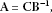(11)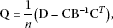(12)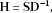(13)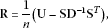(14)

where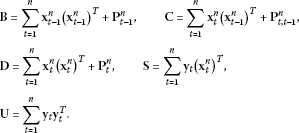The method can be summarized as follows.

1. 1.

Initialize the procedure by selecting starting values for the elements of the parameter set $Θ ( 0 ) ={ A ( 0 ) , H ( 0 ) , Q ( 0 ) , R ( 0 ) }$ and estimate $μ 0$.

2. 2.

(E-step) For $j=1,2,…$ , use the parameter set $Θ ( j − 1 )$ to estimate the smoothed values $x t n$,$P t n$, $P t , t − 1 n$ (equations (8)-(10)) for $t=1,2,…,n$.

3. 3.

(M-step) Calculate a new set of parameters $Θ ( j )$ using equations (11)-(14).

4. 4.

Repeat steps 2 and 3 above until convergence is achieved.

## 5 Simulation results

A 500 Monte Carlo simulation is performed to illustrate the utility of the interval EM algorithm estimate. The observed data are generated according to the second order interval state space model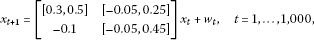(15)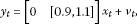(16)

where $w t$ and $v t$ are independent identically distributed (i.i.d.) Gaussian noises such that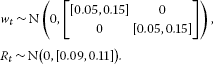This model is a slightly modified version of the one used in . In all simulations, the number of iterations for the EM algorithm is fixed at $J=100$. We used the α values of $α=0:0.1:1$ for the interval estimates. Figure 1 shows a sample of realizations of the minimum, true and maximum observed output data $y t$ respectively, while Figure 2 shows the corresponding interval EM estimate of the output observation. Figure 3 compares the maximum and estimated observed output signals, and Figure 4 shows the minimum and estimated observed output signal. Furthermore, we computed the mean square error (MSE):

$E N = 1 N ∑ t = 1 N ( y t − C x t | t − 1 ) 2$

between the maximum observed and estimated output. The 500 run gave an MSE of 0.0445. A similar computation for the minimum gave an MSE of 0.0447.

## References

1. 1.

Chen G, Wang J, Shieh LS: Interval Kalman filtering. IEEE Trans. Aerosp. Electron. Syst. 1997, 33(1):250–259.

2. 2.

Alefeld G, Herzberger J: Introduction to Interval Computations. Academic Press, San Diego; 1983.

3. 3.

Rohn J: Inverse interval matrix. SIAM J. Numer. Anal. 1993, 3: 864–870.

4. 4.

Bentbib AH: Conjugate directions method for solving interval linear systems. Numer. Algorithms 1999, 21: 79–86. 10.1023/A:1019149111226

5. 5.

Kubica BJ, Malinowski K: Interval random variables and their application in queueing systems with long-tailed service times. SMPS 2006, 393–403.

6. 6.

Chen W, Tan S: Robust portfolio selection using interval random programming. In: FUZZ-IEEE, Korea, 2009, August 20-24 (2009)

7. 7.

Aubin J-P, Frankowska H: Set-Valued Analysis. Birkhäuser, Basel; 1990.

8. 8.

Ekland I, Témam R Classics in Applied Mathematics 28. In Convex Analysis and Variational Problems. SIAM, Philadelphia; 1999.

9. 9.

Jazwinski A: Stochastic Precesses and Filtering Theory. Academic Press, New York; 1970.

10. 10.

Tanizaki H: Nonlinear Filtering: Estimation and Applications. Springer, Berlin; 1996.

11. 11.

Bilms, JA: A gentle tutorial of the EM algorithm and its applications to parameter estimation for Gaussian mixture and hidden Markov models. Technical report TR-97–021, ICSI (1997)

12. 12.

Dempster AP, Laird NM, Rubin DB: Maximum likelihood from uncomplete data via the EM algorithm. J. R. Stat. Soc. B 1977, 39: 1–38.

13. 13.

Shumway RH, Stoffer DS: An approach to time series smoothing and forecasting using the EM algorithm. J. Time Ser. Anal. 1982, 3(4):253–264. 10.1111/j.1467-9892.1982.tb00349.x

14. 14.

Shumway RH, Stoffer DS: Time Series Analysis and Its Applications. Springer, Berlin; 2006.

## Acknowledgements

Dr. O. Al-Gahtani extends his appreciation to the Research Center of Teachers College, King Saud University for funding his work through the research group project No. RGP-TCR-07. The second and third authors would like to thank King Fahd University of Petroleum and Minerals for the excellent research facilities they provide.

## Author information

Authors

### Corresponding author

Correspondence to O Al-Gahtani.

### Competing interests

The authors declare that they have no competing interests.

### Authors’ contributions

The authors have achieved equal contributions to each part of this paper. All authors read and approved the final version of the manuscript.

## Authors’ original submitted files for images

Below are the links to the authors’ original submitted files for images.

## Rights and permissions

Reprints and Permissions

Al-Gahtani, O., Al-Mutawa, J., El-Gebeily, M. et al. The interval versions of the Kalman filter and the EM algorithm. Adv Differ Equ 2012, 172 (2012). https://doi.org/10.1186/1687-1847-2012-172